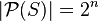## Sunday, February 25, 2007

### Shortcut to Calculating a Power Set (using Java)

This information comes from a question I answered on a message board, and thought it was interesting enough to put in my blog. A power set is written as P(S) and is the set of all subsets of S. For example if S = {a,b,c} then P(S) = {{}, {c}, {b}, {b, c}, {a}, {a, c}, {a, b}, {a, b, c}} Where the number of sets in P is 2 to the power of the number of elements in S:Now consider the binary counting system using 3 digits: 000 001 010 011 100 101 110 111 What you get is all combinations of 0 and 1 for 3 digits, where the number of elements in the 3 digit binary counting system is 2^3. Notice the connection between the binary counting system and power sets yet? Now pretend that each digit of each number in the 3 digit binary counting system indicates whether the corresponding element in the original set is on or off: 000 (a=off, b=off, c=off) {} 001 (a=off, b=off, c=on) {c} 010 (a=off, b=on, c=off) {b} 011 (a=off, b=on, c=on) {b,c} 101 (a=on, b=off, c=on) {a,c} 110 (a=on, b=on, c=off) {a,b} 111 (a=on, b=on, c=on) {a,b,c} By running a binary counter from 0 to 2^number of elements in your set, you can determine the power set of any size set composed of any element. As for data structures you will want to use the java.util.LinkedHashSet, because it maintains insertion order.

```    public static void main(String[] args) {

//construct the set S = {a,b,c}
String set[] = {"a", "b", "c"};

//form the power set
LinkedHashSet myPowerSet = powerset(set);

//display the power set
System.out.println(myPowerSet.toString());

}

/**
* Returns the power set from the given set by using a binary counter
* Example: S = {a,b,c}
* P(S) = {[], [c], [b], [b, c], [a], [a, c], [a, b], [a, b, c]}
* @param set String[]
* @return LinkedHashSet
*/
private static LinkedHashSet powerset(String[] set) {

//create the empty power set
LinkedHashSet power = new LinkedHashSet();

//get the number of elements in the set
int elements = set.length;

//the number of members of a power set is 2^n
int powerElements = (int) Math.pow(2,elements);

//run a binary counter for the number of power elements
for (int i = 0; i < powerElements; i++) {

//convert the binary number to a string containing n digits
String binary = intToBinary(i, elements);

//create a new set
LinkedHashSet innerSet = new LinkedHashSet();

//convert each digit in the current binary number to the corresponding element
//in the given set
for (int j = 0; j < binary.length(); j++) {
if (binary.charAt(j) == '1')
innerSet.add(set[j]);
}

//add the new set to the power set
power.add(innerSet);

}

return power;
}

/**
* Converts the given integer to a String representing a binary number
* with the specified number of digits
* For example when using 4 digits the binary 1 is 0001
* @param binary int
* @param digits int
* @return String
*/
private static String intToBinary(int binary, int digits) {

String temp = Integer.toBinaryString(binary);
int foundDigits = temp.length();
String returner = temp;
for (int i = foundDigits; i < digits; i++) {
returner = "0" + returner;
}

return returner;
} ```
The result for {"a","b","c"} is the following: [[], [c], [b], [b, c], [a], [a, c], [a, b], [a, b, c]] Anything can be put into this list also, with any number of elements. For example {"Cat", "Dog", "Mouse", "Monkey"}: [[], [Monkey], [Mouse], [Mouse, Monkey], [Dog], [Dog, Monkey], [Dog, Mouse], [Dog, Mouse, Monkey], [Cat], [Cat, Monkey], [Cat, Mouse], [Cat, Mouse, Monkey], [Cat, Dog], [Cat, Dog, Monkey], [Cat, Dog, Mouse], [Cat, Dog, Mouse, Monkey]] Sources http://en.wikipedia.org/wiki/Power_set http://www.mathsisfun.com/sets/power-set.html http://en.wikipedia.org/wiki/Combination

#### 7 comments:

Unknown said...

The agreed order of P(S) given S = {a,b,c} is {{}, {a}, {b}, {c}, {a, b}, {a, c}, {b, c}, {a, b, c}}, but not {{}, {c}, {b}, {b, c}, {a}, {a, c}, {a, b}, {a, b, c}}. Proposed ordering can cause difficulties, cuz usually you expect subsets (entries of powerset) to be in the increasing size order...

SAL said...

Thanks for code, just was what I seek.

Eze said...

this only works for sets with at most 32 elements.

Unknown said...

Hey!! thank you very much for taking the time to explain the PowerSet! Your code and explanation is very understandable!!!

Mashhood Syed said...

can you use an array of chars instead of an array of type String?

User enters the size N.

For example can I use char [] alphabetArray = new char [n]

Bill N said...

Thanks for a clear and thorough problem definition and solution. Great job!

Bill N said...

Thanks for a clear and thorough problem definition and solution. Great job!